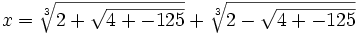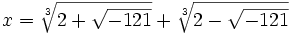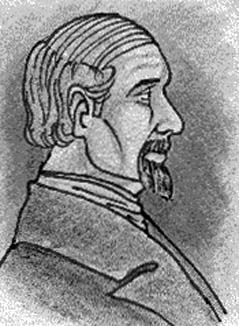## Saturday, December 02, 2006

### Ferrari's Solution to the quartic equation

In today's blog, I go through Lodovico Ferrari's solution of the general quartic equation. In my next blog, I will present a proof of Ferrari's method.

The content in today's blog is taken from Jean-Pierre Tignol's Galois' Theory of Algebraic Equations.

(1) Ferrari started out with a quadratic equation of the following form:

x4 + bx3 + cx2 + dx + e = 0

(2) Then, he borrowed a method from Girolamo Cardano (see here) and set y = x + (b/4) which means x = y - (b/4).

(3) Substituting x = y - (b/4) gives us:

(y - [b/4])4 + b(y - [b/4])3 + c(y - [b/4])2 + d(y - [b/4]]) + e = 0

Working this through (see details here), gives us:

y4 + py2 + qy + r = 0

where:

p = c - 6(b/4)2

q = d - (b/2)c + (b/2)3

r = e - (b/4)c + (b/4)2c - 3(b/4)4

(4) Now if we move the q and r term over we get:

y4 + py2 = -qy -r

This helps us because if we add (p/2)2 to both sides, we can complete the square to get:

(y2 + p/2)2 = -qy -r + (p/2)2

(5) Now, if we add a value u to our square, we get the following:

(y2 + p/2 + u)2 = -qy -r + (p/2)2 + 2uy2 + pu + u2

This may not appear to help us but it gives us a possibility for completing the square on the other side.

(6) For example, if we could find a value u such that:

-qy -r + (p/2)2 + 2uy2 + pu + u2 = ([√2uy - q/(2√2u)]2

Then we have found a solution (see step #11 if this point is not clear)

(7) Now:

([√2uy - q/(2√2u)]2 = 2u2y2 - 2*(√2uy)(q/(2√2u)) + q2/(8u) =

= 2u2y2 - q/u + q2/(8u)

(8) So this equation works only if:

-qy -r + (p/2)2 + 2uy2 + pu + u2 = 2uy2 - qy + q2/(8u)

That is if:

-r + (p/2)2 + pu + u2 = q2/(8u)

(9) But if we multiply 8u to all sides and then subtract by q2, we get:

8u3 + 8pu2 + (2p2 - 8r)u - q2 = 0

(10) This is the general cubic equation which we can solve using Cardano's formula so we know that u can take on this value.

(11) This then gives us:

(y2 + p/2 + u)2 = ([√2uy - q/(2√2u)]2.

(12) This can we solve using the quadratic equation. The four solutions can be found from:

(y2 + p/2 + u)2 = ± [√2uy - q/(2√2u)]

I should probably note that we assumed that u ≠ 0. I will talk about the situation were u = 0 in my next blog where I provide a proof for Ferrari's solution.

References

## Friday, December 01, 2006

### Bombelli and the invention of complex numbers

Rafael Bombelli was the first to propose the idea of complex numbers.

Bombelli wrote about imaginary numbers in his very influential book Algebra which was published in 1572.

Bombelli based his argument for their usefulness on Girolamo Cardano's solution for the cubic equation. Consider the following equation:

x3 = 15x + 4.

This is an example of the depressed cubic so we can use del Ferro/Tartaglia solution (see here):

b=-15
c=4

with:This then gives us:So that:which simplies to:Cardano had considered such forms "irreducible" and had ignored them. Bombelli noticed that this wasn't quite right. For example, x3 = 15x + 4 is solved when x=4.

Bombelli proposed what he called a "wild thought". What if the term -1 could be used mathematically so that the above value could be reduced to a number of the form: a+b√-1.

Bombelli proposed mathematical rules for allowing such numbers:

b1-1 + b2-1 = (b1 + b2)√-1

(b1-1)*(b2-1) = (-1)b1b2

and finally if:

a + bi = c + di, then a=c and b=d.

Then, using these assumptions, here's what he found:

(1) Assume that:

( a + b√-1)3 = 2 + √-121

(2) We note that (m + n)3 = m3 + n3 + 3mn(m + n) since:

(m + n)3 = m3 + 3m2n + 3mn2 + n3 = m3 + n3 + 3mn(m + n)

(3) Let m = a, n=b√-1 which gives us:

( a + b√-1)3 = a3 + b3(-1)√-1 + 3(a)(b√-1)(a + b√-1) =

=a3 - b3-1 + 3a2(b√-1) + 3ab2(-1) =

= a(a2 -3b2) + b(3a2 - b2)√-1.

(4) Now solving for ( a + b√-1)3 = 2 + √-121 gives us:

a(a2 -3b2) = 2

b(3a2 - b2) = 11

(5) Since 2,11 are primes, we know that in both cases, one of the two factors must be 1.

If we assume that a=2,b=1, then we see that:

22 - 3(12) = 1
3*22 - 12 = 11

(6) So that:

(2 + √-1)3 = 2 + √-121.

(7) And using the same reasoning, we see that:

(2 - √-1)3 = 2 - √-121.

(8) If we assume that his reasoning is sound, we now find that:reduces to:

x = (2 + √-1) + (2 - √-1) = 4

In this way, the theory of complex numbers was first presented by Bombelli and was eventually accepted by mathematicians.

References

## Monday, November 27, 2006

### Rafael BombelliRafael Bombelli was born in 1526 in Bologna. His father, Antonio Mazzoli, changed his name to Bombelli in order to avoid the reputation of the Mazzoli family. The Mazzoli family had once been very powerful and influential in Bologna. This all changed when Pope Julius II came to power. In 1506, the ruling family, the Bentivoglios, were exiled. In 1508, there was an effort to take back Bologna which failed. Mazzoli's grandfather was among those captured and executed. Later, Antonio Bombelli, was able to make his way back to Bologna as a wool merchant.

Rafael was the eldest of six children of Antonio Bombelli. He did not go to the university but learned mathematics as an engineer. He took a deeper interest in mathematics and managed to read many of important math works of his day.

At this time, there was great publicity regarding the controversy around the solution of the cubic equation between Girolamo Cardano and Nicolo Fontana Tartaglia.
It is clear that Bombelli came to read Cardano's Ars Magna and also that he closed followed the debate between Tartaglia and Lodovico Ferrari in Milan in 1548.

We know that in 1549, Bombelli was tasked by Alessandro Rufini to work on a major engineering project to reclaim marshlands. He continued to work on this project unil 1555 when the work there was put on hold.

It was at this time, during the interruption of work, that Bombelli began work on a math book on algebra. He believed that the only work which delved deeply into the subject, Cardano's work, was too difficult and subtle for a majority of mathematicians. He sought to provide a clearer and more accessible version.

In 1560, Bombelli resumed the major engineering project. The project was deemed a giant success and afterwards, Bombelli found himself with a reputation as a very talented hydraulic engineer. He was now tasked to do other very important projects.

One time, when he was traveling in Rome, he learned about Diophantus's Arithmetica. He had not yet finished his intended book on algebra so his reading of Diophantus had a great impact on his math project. Bombelli then published the first three parts of his algebra in 1572.

Bombelli's Algebra is one of the most important and influential works ever published. It is a thorough and in depth analysis of the state of algebra. In it, he introduces the idea of complex numbers for the first time. Others had noticed the idea of negative numbers and the possibility of negative roots that appear in Cardano's cubic equation but it was Bombelli who first defended them as worthy of study in themselves.

Bombelli proposed a mathematical notation that was a significant advancement at the time.He also proposes rules for addition, subtraction, and multiplication of complex numbers. Most importantly, he showed how reasoning about complex numbers using Cardano's cubic equation could lead to real values. I will go into more detail about this in my next blog.

Bombelli had intended to publish two more parts but he did not finish them. He died in 1572. The last two parts were later found and published in 1929. Today, Bombelli is considered the inventor of complex numbers.

References